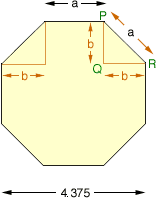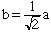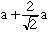Subject: Octagon problem How do I figure the length of the sides of an octagon when all I know is the diameter (4.375). Craig Middle Parent Thank you, Hi Craig, For the regular octagon in the diagram below let a be the length of each side. From the symmetry, the triangle PQR is a right triangle with two sides of equal length. I have called this length b.From the theorem of Pythagoras, b2 + b2 = a2 and henceAgain looking at the diagram 4.375 = b + a + b =Now you can solve for a. Cheers, Penny Go to Math Central